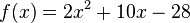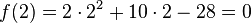# Polynomial root facts for kids

Kids Encyclopedia Facts

The root of a polynomial f is a value for x where the polynomial value f(x) is zero.

For example, if the polynomial$f(x) = 2x^2 + 10x - 28$ then$f(2) = 2\cdot 2^2 + 10\cdot 2 - 28=0$, so 2 is a root of this polynomial.

In fact, it can be seen that also -7 is a root of this polynomial.Polynomial root Facts for Kids. Kiddle Encyclopedia.